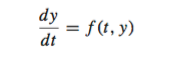## Posts

Showing posts from June, 2016

### Solving Differential Equations:One step MethodsA lot of problems in science and engineering involve solving differential equations pendulums, RLC circuits, diffusion and so on. An ordinary  differential equation can be expressed as:  Generally solution of this equation is of the form: Here ' phi ' is the increment function or slope and h is the step size.  Thus, an estimate of slope is used to extrapolate the new value y(i+1) when the previous value y(i) is known, over a distance h .  The methods which are based on above equation are called one step methods . They all only differ by the definition of increment function. Solved Example: Calculate y(2) for  in the interval t=[0,2]. Given y(0)=1, for Solution: Consider h=1, here Using Euler Method:

### Separate Axis TheoremIntroduction Separate Axis Theorem (SAT) is a method to determine whether two convex shapes are intersecting or not. The theorem states that, “Given two convex shapes there exists a line onto which their projections will be separate if and only if they are not intersecting”.   It is the most commonly used method for collision detection.   SAT also returns the minimum penetration vector, or minimum translation vector, the shortest distance that the colliding object can be moved in order to no longer be colliding. The basic idea is imagine you have two convex shapes, let us consider two triangles as given in fig 1. We shine light on them from different angles and observe shadows on the wall perpendicular to the direction of light, if doing this work around the shapes we do not find a gap in the shadows, then the objects are colliding. But, if we are able find a gap, then they are not intersecting/colliding. Algorithm:  The first question that arises while implementing SAT

### RAM : Different Speeds Different SizesHave you ever needed to replace the RAM of your desktop or Laptop? Have you wondered what if you add two RAM off different speeds, say one at 1600 Mhz and other 1330 MHz? Or if you mixed the sizes one RAM of 8GB and another of 2GB? The worst case scenario, system may not boot!! The system may not be able to come to the optimized value of speed or size. The best scenario, system optimize the speed to the lowest configuration (actually it is more like two resistances in parallel, the resultant is lowest than the two). Shown below is Memory configuration of Mac Mini, when two 8GB DDR3 RAMs one of 1600MHz (Crucial) and other of 1330 MHz (Corsair) is put, see the net speed 1067Mhz, lower than the lowest. Exactly similar thing will happen if the two memories are different. You will not get the net result of 10GB memory (8+2), because OS treats the two equal, while paging, hence even though it will show 8GB + 2GB but the memory used will be <4GB. Verdict: Do not mix RAM sizes or speeds. R